Previous Page | Next Page

 The POWER Procedure
 Determining Required Sample Size for a Two-Sample t Test

In this example you want to compare two physical therapy treatments designed to increase muscle flexibility. You need to determine the number of patients required to achieve a power of at least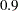to detect a group mean difference in a two-sampletest. You will use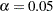(two-tailed).

The mean flexibility with the standard treatment (as measured on a scale of 1 to 20) is well known to be about 13 and is thought to be between 14 and 15 with the new treatment. You conjecture three alternative scenarios for the means:

1.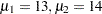2.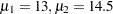3.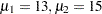You conjecture two scenarios for the common group standard deviation:

1.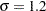2.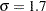You also want to try three weighting schemes:

1. equal group sizes (balanced, or 1:1)

2. twice as many patients with the new treatment (1:2)

3. three times as many patients with the new treatment (1:3)

This makes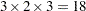scenarios in all.

Use the TWOSAMPLEMEANS statement in the POWER procedure to determine the sample sizes required to give 90% power for each of these 18 scenarios. Indicate total sample size as the result parameter by specifying the NTOTAL= option with a missing value (.). Specify your conjectures for the means by using the GROUPMEANS= option. Using the "matched" notation (discussed in the section Specifying Value Lists in Analysis Statements), enclose the two group means for each scenario in parentheses. Use the STDDEV= option to specify scenarios for the common standard deviation. Specify the weighting schemes by using the GROUPWEIGHTS= option. You could again use the matched notation. But for illustrative purposes, specify the scenarios for each group weight separately by using the "crossed" notation, with scenarios for each group weight separated by a vertical bar (|). The statements that perform the analysis are as follows:

```proc power;
twosamplemeans
groupmeans   = (13 14) (13 14.5) (13 15)
stddev       = 1.2 1.7
groupweights = 1 | 1 2 3
power        = 0.9
ntotal       = .;
run;
```

Default values for the TEST=, DIST=, NULLDIFF=, ALPHA=, and SIDES= options specify a two-sidedtest of group mean difference equal to 0, assuming a normal distribution with a significance level of= 0.05. The results are shown in Figure 68.4.

Figure 68.4 Sample Size Analysis for Two-Sample t Test Using Group Means
The POWER Procedure
Two-sample t Test for Mean Difference

Fixed Scenario Elements
Distribution Normal
Method Exact
Group 1 Weight 1
Nominal Power 0.9
Number of Sides 2
Null Difference 0
Alpha 0.05

Computed N Total
Index Mean1 Mean2 Std Dev Weight2 Actual Power N Total
1 13 14.0 1.2 1 0.907 64
2 13 14.0 1.2 2 0.908 72
3 13 14.0 1.2 3 0.905 84
4 13 14.0 1.7 1 0.901 124
5 13 14.0 1.7 2 0.905 141
6 13 14.0 1.7 3 0.900 164
7 13 14.5 1.2 1 0.910 30
8 13 14.5 1.2 2 0.906 33
9 13 14.5 1.2 3 0.916 40
10 13 14.5 1.7 1 0.900 56
11 13 14.5 1.7 2 0.901 63
12 13 14.5 1.7 3 0.908 76
13 13 15.0 1.2 1 0.913 18
14 13 15.0 1.2 2 0.927 21
15 13 15.0 1.2 3 0.922 24
16 13 15.0 1.7 1 0.914 34
17 13 15.0 1.7 2 0.921 39
18 13 15.0 1.7 3 0.910 44

The interpretation is that in the best-case scenario (large mean difference of 2, small standard deviation of 1.2, and balanced design), a sample size of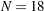(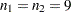) patients is sufficient to achieve a power of at least 0.9. In the worst-case scenario (small mean difference of 1, large standard deviation of 1.7, and a 1:3 unbalanced design), a sample size of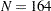(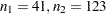) patients is necessary. The Nominal Power of 0.9 in the "Fixed Scenario Elements" table represents the input target power, and the Actual Power column in the "Computed N Total" table is the power at the sample size (N Total) adjusted to achieve the specified sample weighting exactly.

Note the following characteristics of the analysis, and ways you can modify them if you want:

• The total sample sizes are rounded up to multiples of the weight sums (2 for the 1:1 design, 3 for the 1:2 design, and 4 for the 1:3 design) to ensure that each group size is an integer. To request raw fractional sample size solutions, use the NFRACTIONAL option.

• Only the group weight that varies (the one for group 2) is displayed as an output column, while the weight for group 1 appears in the "Fixed Scenario Elements" table. To display the group weights together in output columns, use the matched version of the value list rather than the crossed version.

• If you can specify only differences between group means (instead of their individual values), or if you want to display the mean differences instead of the individual means, use the MEANDIFF= option instead of the GROUPMEANS= option.

The following statements implement all of these modifications:

```proc power;
twosamplemeans
nfractional
meandiff     = 1 to 2 by 0.5
stddev       = 1.2 1.7
groupweights = (1 1) (1 2) (1 3)
power        = 0.9
ntotal       = .;
run;
```

Figure 68.5 shows the new results.

Figure 68.5 Sample Size Analysis for Two-Sample t Test Using Mean Differences
The POWER Procedure
Two-sample t Test for Mean Difference

Fixed Scenario Elements
Distribution Normal
Method Exact
Nominal Power 0.9
Number of Sides 2
Null Difference 0
Alpha 0.05

Computed Ceiling N Total
Index Mean Diff Std Dev Weight1 Weight2 Fractional N Total Actual Power Ceiling N
Total
1 1.0 1.2 1 1 62.507429 0.902 63
2 1.0 1.2 1 2 70.065711 0.904 71
3 1.0 1.2 1 3 82.665772 0.901 83
4 1.0 1.7 1 1 123.418482 0.901 124
5 1.0 1.7 1 2 138.598159 0.901 139
6 1.0 1.7 1 3 163.899094 0.900 164
7 1.5 1.2 1 1 28.961958 0.900 29
8 1.5 1.2 1 2 32.308867 0.906 33
9 1.5 1.2 1 3 37.893351 0.901 38
10 1.5 1.7 1 1 55.977156 0.900 56
11 1.5 1.7 1 2 62.717357 0.901 63
12 1.5 1.7 1 3 73.954291 0.900 74
13 2.0 1.2 1 1 17.298518 0.913 18
14 2.0 1.2 1 2 19.163836 0.913 20
15 2.0 1.2 1 3 22.282926 0.910 23
16 2.0 1.7 1 1 32.413512 0.905 33
17 2.0 1.7 1 2 36.195531 0.907 37
18 2.0 1.7 1 3 42.504535 0.903 43

Note that the Nominal Power of 0.9 applies to the raw computed sample size (Fractional N Total), and the Actual Power column applies to the rounded sample size (Ceiling N Total). Some of the adjusted sample sizes in Figure 68.5 are lower than those in Figure 68.4 because underlying group sample sizes are allowed to be fractional (for example, the first Ceiling N Total of 63 corresponding to equal group sizes of 31.5).Previous Page | Next Page | Top of Page